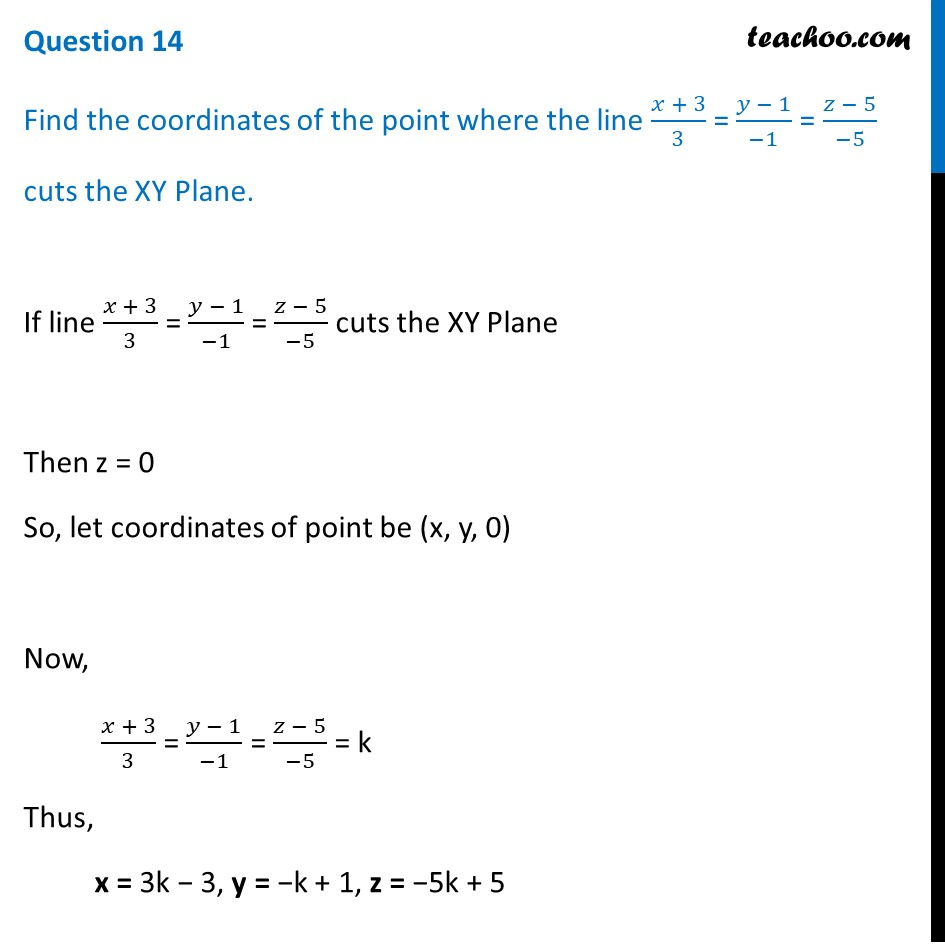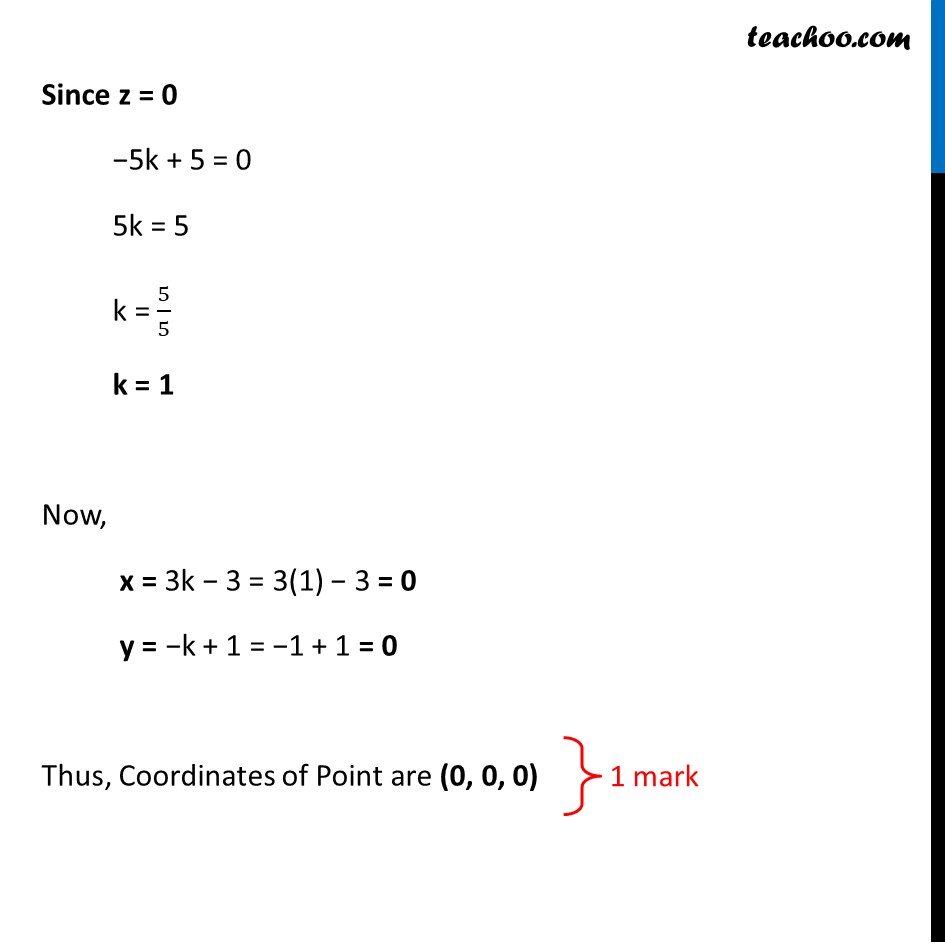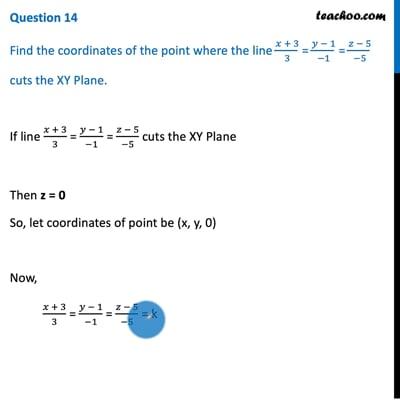CBSE Class 12 Sample Paper for 2021 Boards

Class 12
Solutions of Sample Papers and Past Year Papers - for Class 12 Boards

## Find the coordinates of the point where the line (x+3)/3 = (y-1)/(-1) = (z-5)/(-5) cuts the XY Plane.This video is only available for Teachoo black users

Note : This is similar to Example 30 of NCERT – Chapter 11 Class 12 Three Dimensional Geometry

Check the answer here https:// www.teachoo.com /3586/756/Example-30---Find-point-where-line-crosses-the-XY-plane/category/Examples/

Introducing your new favourite teacher - Teachoo Black, at only ₹83 per month

### Transcript

Question 14 Find the coordinates of the point where the line (𝑥 + 3)/3 = (𝑦 − 1)/(−1) = (𝑧 − 5)/(−5) cuts the XY Plane. If line (𝑥 + 3)/3 = (𝑦 − 1)/(−1) = (𝑧 − 5)/(−5) cuts the XY Plane Then z = 0 So, let coordinates of point be (x, y, 0) Now, (𝑥 + 3)/3 = (𝑦 − 1)/(−1) = (𝑧 − 5)/(−5) = k Thus, x = 3k − 3, y = −k + 1, z = −5k + 5 Since z = 0 −5k + 5 = 0 5k = 5 k = 5/5 k = 1 Now, x = 3k − 3 = 3(1) − 3 = 0 y = −k + 1 = −1 + 1 = 0 Thus, Coordinates of Point are (0, 0, 0)